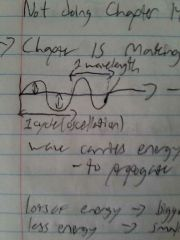• Shuffle
Toggle On
Toggle Off
• Alphabetize
Toggle On
Toggle Off
• Front First
Toggle On
Toggle Off
• Both Sides
Toggle On
Toggle Off
Toggle On
Toggle Off
Front

## Card Range To Study

throughPlay buttonPlay buttonProgress

1/22

Click to flip

Use LEFT and RIGHT arrow keys to navigate between flashcards;

Use UP and DOWN arrow keys to flip the card;

H to show hint;

 What is one wavelength?One oscillation/cycle in a wave. What is the term for how tall a wave is? Amplitude What is the term for one complete oscillation of 1 cycle? Time period (T) What is the formula for frequency? What is the unit? f = 1/T where 1 is the number of oscillations. the unit is Hertz Will water be dragged along with a wave? No, it will merely flow. What is the formula for speed of a wave? Speed of wave = frequency * wavelength V = fλ Longitudinal and transverse are two types of what? What are they based on? They are waves based on how the wave propogates through in the medium. What is the unit of measurement for wavelengths? m/s Which type of wave is when the medium moves up and down as the wave passes by? What are some examples? Transverse waves. Examples include water, light, and instrument Which type of wave is when the medium gets compressed and relaxed as the wave passes by? What are some examples? Longitudinal wave. Examples include sound, wave on a slinky. What kind of waves occur when a wave reflects back on itself? Standing waves. What is it called when a standing wave intersects with the medium? A node What is it called when a crest of a standing wave is met? An anti node. How many waves is the first harmonic? What is the formula? What is the letter for the first harmonic? 1/2 wave L = λ/2 f What is the fundamental frequency? The first harmonic. How many waves is the second harmonic. What is the formula for the second harmonic. What is the letter for the second harmonic? It is one wave L = λ. 2f How many waves is the third harmonic? What is the formula for the third harmonic? What is the letter for the third harmonic? L = 1.5λ or (3/2)λ 3f Do wavelength and frequency work inversely towards each other? Yes, higher wavelength means lower frequency and vice versa Suppose that water waves coming into a dock have a velocity of 1.2 m/s and a wavelength of 2.4m. With what frequency do these waves meet the dock? .05 f=V/λ Suppose that water waves have a wavelength of 4.1 m and a period of 2.3 s. What is the velocity of these waves? f = 1/2.3 (f=1/T) V=fλ The length of two wavelengths is 8 m. a) what is the wavelength of the wave?b) if the frequency is 3Hz, what is the wave speed? a) 8 = 2λ, 4 = λb) V = fλ, V = 3*4 A string with a length of 0.8 m is fixed at both ends.a. What is the longest possible wavelength for the traveling waves that can interfere to form a standing wave on this string?b. If waves travel at the speed of 120 meters per second on this string what is a frequency associated with this longest wavelength? A. The first harmonic is the longest, so L = λ/2 .8 * 2 = 1.6 mB. V=fλ 120m/s = 1.6 m * f, f = 75Hz